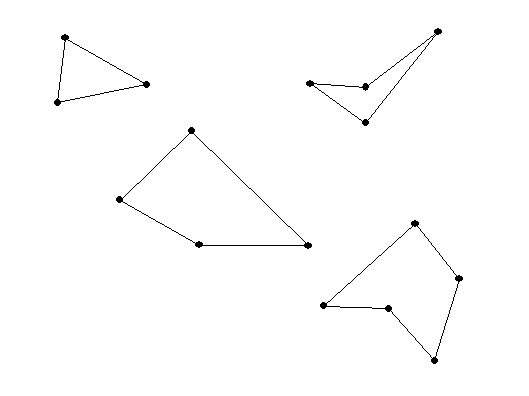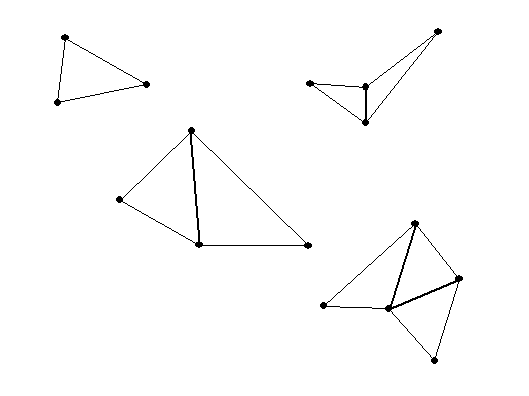Thursday, January 27

Puzzles and Polygons
• Dissections, cut and paste methods of measurement.
• Cutting and reassembling polygons.

• Cutting and reassembling polygons.

Tangoes: a commercial game based on tangrams
Tapestry Project from previous Math 103 students.

Cutting and reassembling polygons.

Convex:    Any two points in the figure have a line segment connecting them. If that line segment is always  inside the figure, then the figure is called "convex".

Making Dissection Puzzles:

Dissection of the plane--- Tilings of the plane:
Tessellations

• Activity: Tile plane with playing cards.
• Preview ... Possible tiles and tilings.
• One Regular Convex Polygon.
• Two or more Regular Convex Polygons.
• Other Polygon Tilings.
• Non polygonal tilings.

• More on measurements of angles and areas of polygons.
•
REVIEW: Recall our previous discussions
for a polygon with n sides.

When n = 3 this is a triangle, n=4, a quadrilateral, or when n= 5, a pentagon.The sum of the measures of the interior angles of a triangle is 180 degrees.
Review Question:What about a quadrilateral? and a pentagon?  or an n sided polygon  ( an "n -gon")?From the figure we saw that for a quadrilateral (n =4), which can be dissected into two triangles,
the sum is 2*180= 360 degrees.
And for a pentagon (n=5) which can be dissected into 3 triangles, the sum is 3*180=540 degrees.

In general: the sum of the interior angles in a n sided polygon is
(n-2) *180 degrees.

A regular  polygon is a polygon where the sides are all of equal length and the angles are all congruent (or of equal measure).

Review Question: what is the measure of an individual angle in a regular polygon with n sides?

For a triangle, the individual angle is 180/3 = 60 degrees.
For a square, the individual angle is  360/4=90 degrees.
For a regular pentagon.... 3*180/5 = 540/5 =108  degrees.

Now for a HEXAGON (6 sides) the sum of the angles is 720 degrees.
So ... for a REGULAR HEXAGON

the individual angle is  4*180/6 =720/6 =120 degrees.

In general: The individual angle for a regular polygon with n sides is (n-2)*180/n degrees.
This can be expressed in other ways using algebra:
(n-2)*180/n = [180 n - 360] / n = 180 - 360/n.

 name of polygon degrees of the interior  measure of each angle 360 degrees divided  by # in Column 2 equilateral triangle 3 60 360 / 3 = 120 square 4 90 360/4= 90 regular pentagon 5 3*180/5= 108 360/5= 72 regular hexagon 6 4*180/6=120 360/6= 60 regular heptagon 7 5*180/7 360/7 regular octagon 8 6*180/8=135 360/8 = 45 regular dodecagon 12 10*180/12=1800/12=150 360/12=30

• Dissection of the plane--- Tilings of the plane.

• One polygonal Tile: Quadrilateral Activity. (related to Assignment due next class!)
• An on-line tool for making tilings of the plane
• Regular and semiregular Tilings of the plane.
• A tiling is a regular tiling if(i)it has a single tile shape that is a regular polygon and (ii) the vertices and edges of the tiles coincide (no overlapping edges)
• A tiling is a semi-regular tiling if (ii) each tile shape is a regular polygon, (ii) the vertices and edges of the tiles coincide (no overlapping edges) and (iii) every vertex has the same polygon types arranged around it.
• Naming tilings (Math Forum)
• The numbers represent the number of sides in the poygons.
• The order indcates the order in which the poygons are arranged about a vertex.
• Local considerations about a vertex. The sum of the angles must equal 360 degrees.
• 4.1  Ex. 3. How many tiles around single vertex: 3,4,5,6,7,8?...
• How can there be 6? Only one way: 3-3-3-3-3-3   (6*60= 360)
• Why not 7? If 7 or more tiles aroung a single vertex the sum of the angles must exceed 360 degrees.
• 4.1  Ex. 4. Can there be 4 different tiles around a single vertex? Not if they are all different since 60+90+108+120 = 378 >360.
• 4.1  Ex. 5. If there are 4 tiles around a vertex then ....At least two of the tiles must have the same number of sides.
• Arithmetic for vertices.

• Example: Three regular polygons about a vertex with n , k, and p sides.

(180 - 360/n) + (180 - 360/k) + (180 - 360/p) = 360

3*180 -360( 1/n+1/k+1/p)= 2*180

1*180 = 360( 1/n+1/k+1/p)

SO....
180/360 = 1/n + 1/k + 1/p  or

1/n + 1/k + 1/p=1/2

So, for example, n=3, k=4 and p= 5 is not possible since

1/3+1/4+1/5 >1/2.

Number of polygons
around a vertex
Equation for angle sum = 360 Equivalent Arithmetic equation Solutions to the arithmetic equations.
3: n , k, p 180 - 360/n+180 - 360/k+180 - 360/p = 360 1/n+1/k+1/p =1/2  6 6 6 5 5 10 4 5 20 4 6 12 4 8 8 3 7 42 3 8 24 3 9 18 3 10 15 3 12 12

4:  n, k, p, z 180 - 360/n+180 - 360/k+180 - 360/p 180 - 360/z = 360 1/n+1/k+1/p +1/z =2/2 =1  4 4 4 4 3 3 4 12 3 3 6 6 3 4 4 6

5:  n, k, p, z, w 180 - 360/n+180 - 360/k+180 - 360/p+180 - 360/z+180 - 360/w = 360 1/n+1/k+1/p +1/z+1/w =3/2  3 3 3 3 6 3 3 3 4 4

• Local consideration about a polygon:
• Examples: If there is an equilateral triangle involved with 2 other polygons, then the other two ploygons must have the same number of sides. Because: if there were two different polygons aroung the triangle, then on vertex would not have all three polygons sharing that vertex. (such as 3-10-15)
• Similar considerations can eliminate tilings using a single pentagon and two other distinct tiles. (such as 4-5-20).
• Semiregular Tilings: global results!
Look at the results using wingeometry.
• Student lesson (Math Forum) a place for further explorations on-line.